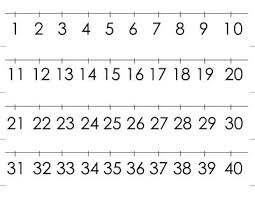# Difference 13903

The sum of two integers is three, and their difference is 7. Determine the unknown numbers.

a =  5
b =  -2

### Step-by-step explanation:

a+b=3
a-b =7

a+b = 3
a-b = 7

Row 2 - Row 1 → Row 2
a+b = 3
-2b = 4

b = 4/-2 = -2
a = 3-b = 3+2 = 5

a = 5
b = -2

Our linear equations calculator calculates it.Did you find an error or inaccuracy? Feel free to write us. Thank you!

Tips for related online calculators
Do you have a linear equation or system of equations and looking for its solution? Or do you have a quadratic equation?